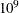### Problem Statistics

Optimizers can encounter difficulty when solving poorly formulated models. Information about data magnitude provides a simple gauge to determine how well a model is formulated. For example, a model whose constraint matrix contains one very large entry (on the order of) can cause difficulty when the remaining entries are single-digit numbers. The PRINTLEVEL=2 option in the OPTMODEL procedure causes the ODS table ProblemStatistics to be generated when the LP solver is called. This table provides basic data magnitude information that enables you to improve the formulation of your models.

The example output in Figure 6.3 demonstrates the contents of the ODS table ProblemStatistics.

Figure 6.3: ODS Table ProblemStatistics

The OPTMODEL Procedure

Problem Statistics
Number of Constraint Matrix Nonzeros 6
Maximum Constraint Matrix Coefficient 3
Minimum Constraint Matrix Coefficient 1
Average Constraint Matrix Coefficient 2.1666666667

Number of Objective Nonzeros 3
Maximum Objective Coefficient 1
Minimum Objective Coefficient 1
Average Objective Coefficient 1

Number of RHS Nonzeros 2
Maximum RHS 1
Minimum RHS 1
Average RHS 1

Maximum Number of Nonzeros per Column 2
Minimum Number of Nonzeros per Column 2
Average Number of Nonzeros per Column 2

Maximum Number of Nonzeros per Row 3
Minimum Number of Nonzeros per Row 3
Average Number of Nonzeros per Row 3